## 6-colourings of subsets of the plane

There has been further recent activity on the Chromatic Number of the Plane problem, with an eleventh research thread being spawned. Philip Gibbs has been able to 6-colour a large disc (with diameter slightly greater than 4), and Aubrey de Gray has remarked that it can be enlarged slightly further still: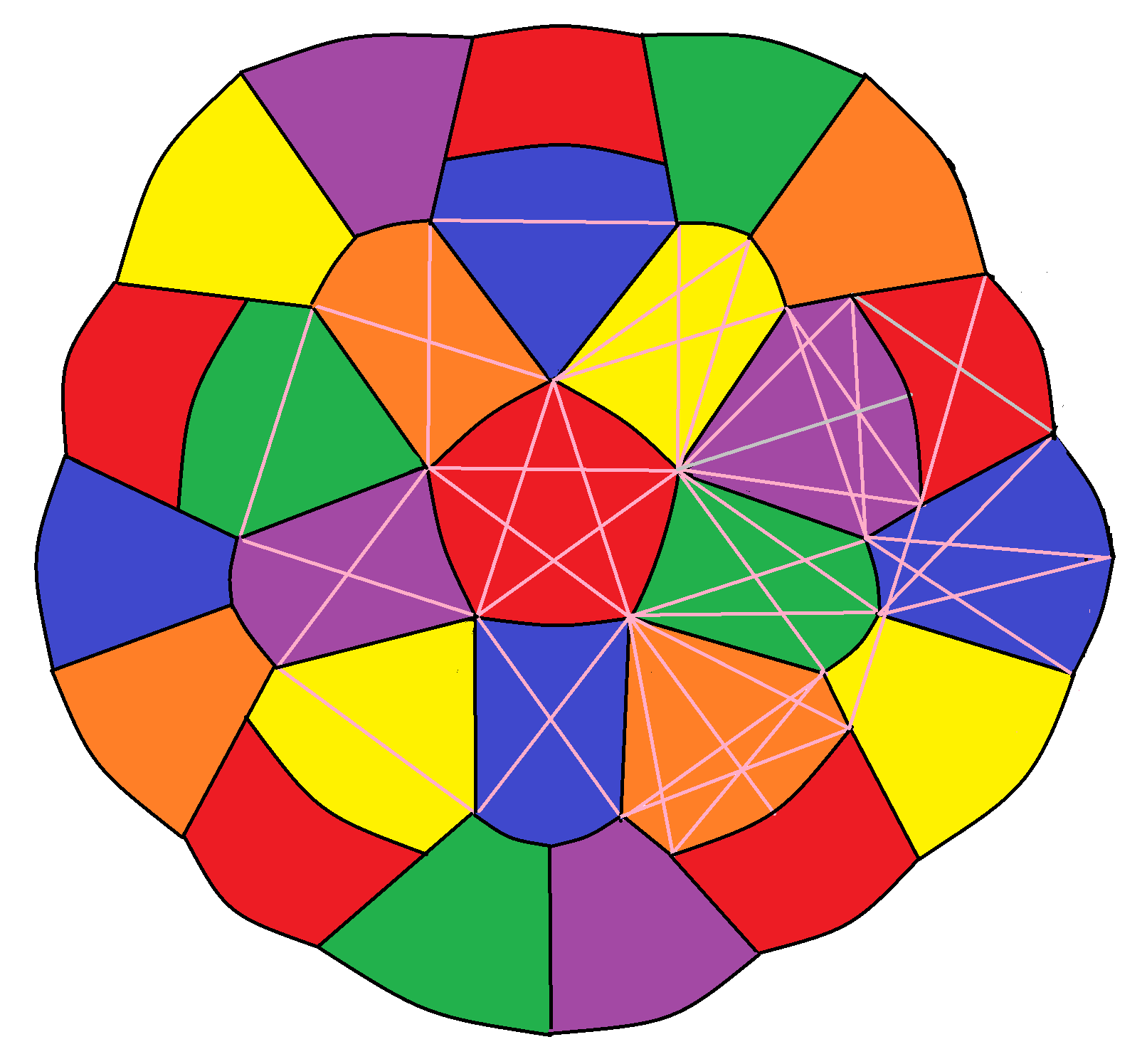An infinite strip of width$\sqrt{3} + \frac{1}{2} \sqrt{15}$ can similarly be 6-coloured in a relatively simple way.

### What about the whole plane?

Interestingly, it has been shown that any tile-based 6-colouring of the plane is critical in the sense that the maximum diameter of any tile must be equal to the minimum separation between similarly-coloured tiles; there is no room for manoeuvre. Moreover, this means that it is insufficient to simply specify the colours of the tiles themselves; it is necessary to also colour the (measure-0) vertices and edges where they meet!

More updates as events warrant…

Posted in Uncategorized | 2 Comments

## Atiyah’s problem

At the Heidelberg Laureate Forum three years ago, I took lots of selfies with Fields medallists, Abel prizewinners and Turing laureates. This included having a dinner in a castle with Leonard Adleman, pioneer of asymmetric cryptography: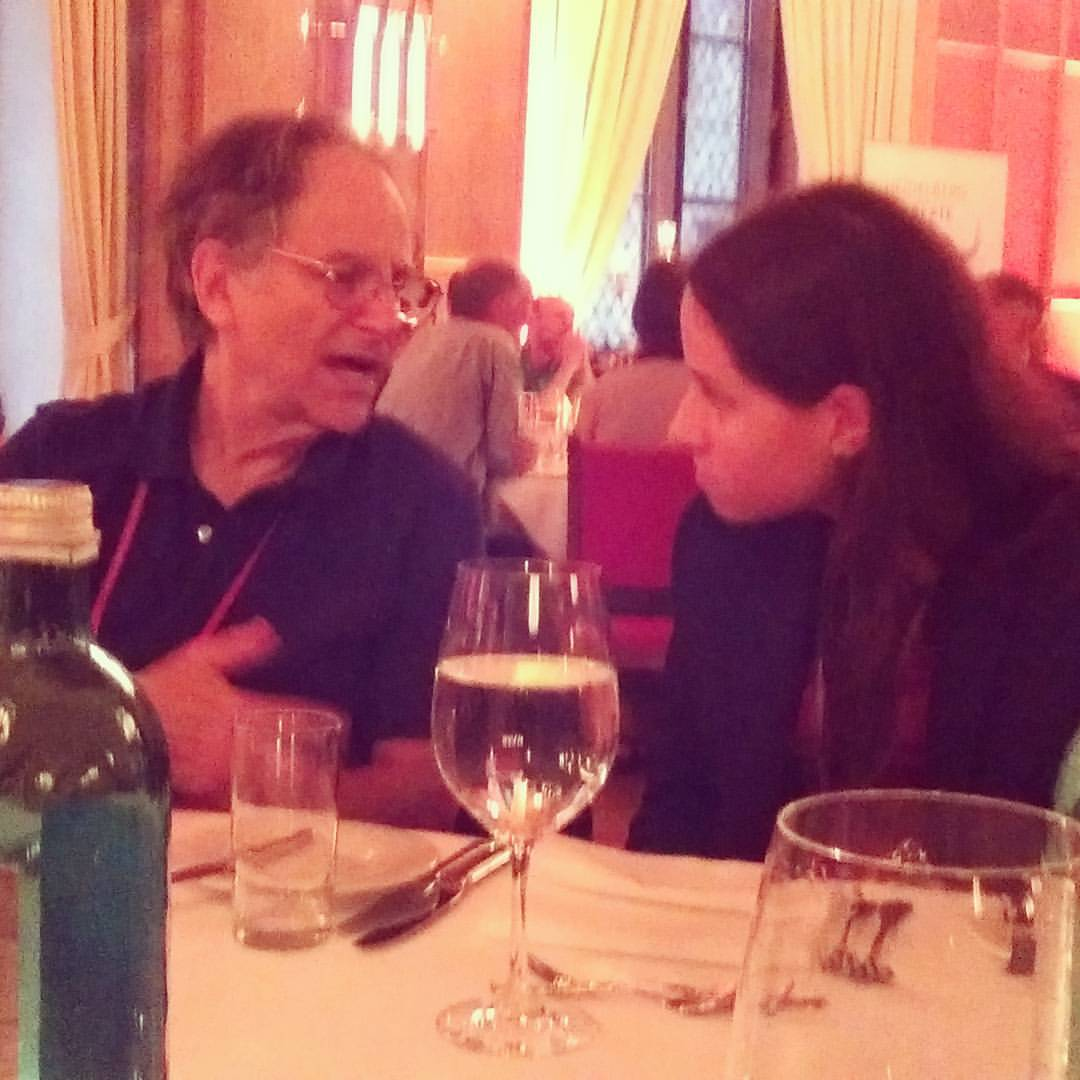…and Endre Szemeredi of regularity lemma fame……and Louis Nirenberg……and, last but certainly not least, enjoyed sparkling Riesling in a Bavarian brewery with Michael Atiyah: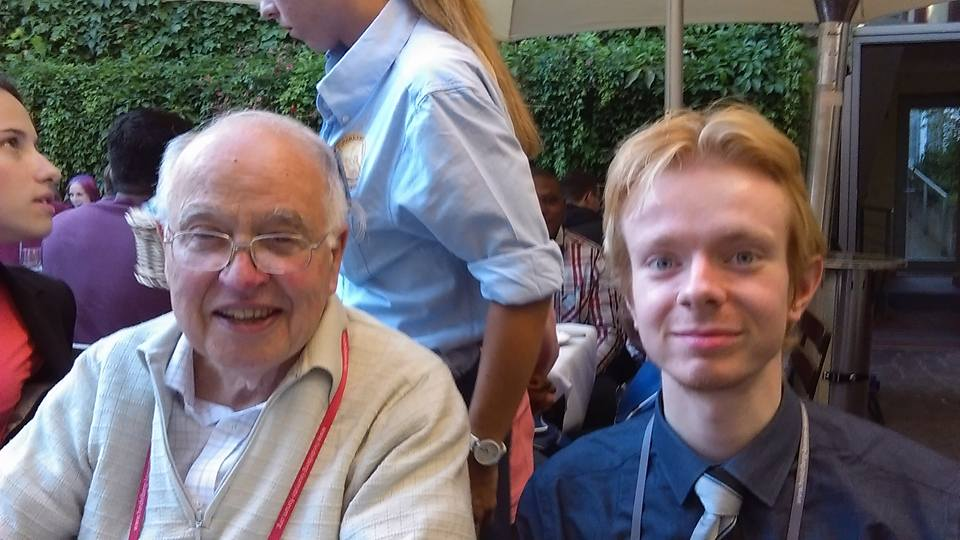He proceeded to summon several of us into a room, wherein he posed a rather interesting problem and offered a reward for its solution:

Consider n distinct points,$x_1, \dots, x_n$ in the three-dimensional unit ball. Let the ray (half-line) from$x_i$ through$x_j$ meet the boundary of the ball at$z_{ij}$, viewed as a complex number on the Riemann sphere. We define the monic polynomials$P_i(t) := \prod_{j \neq i} (t - z_{ij})$ whose roots are given by the projections of the remaining points onto the sphere.

Prove that these n polynomials are linearly independent.

If we consider the determinant of the matrix M formed by the coefficients of these polynomials, we get a degree-½n(n−1) homogeneous polynomial in the n(n−1) roots. This determinant can be seen to be invariant under adding a constant to all roots, but it is not scale-invariant because the degree is nonzero. This can be amended by dividing by a normalising constant, yielding a rational function δ:$\delta := \det M / \prod_{i < j} (z_{ij} - z_{ji})$

Note that δ is not only scale- and translation-invariant, but also is invariant under simultaneously replacing all roots by their reciprocals. This means that δ is invariant under the entirety of the Möbius group, which corresponds naturally to the group of orientation-preserving projective transformations fixing the unit ball. Since δ is dimensionless, it is reasonable to conjecture the following stronger problem:

Prove that |δ| ≥ 1.

Apparently an acquaintance of Atiyah proved this for up to 4 points by symbolic manipulation in a computer algebra package, and experimentally verified that it appears to hold in much higher dimensions.

Interestingly, if one of the points$x_i$ is on the boundary of the unit ball, it can be seen that deleting it does not alter the value of δ. (Hint: since we have so much invariance, it suffices to check this at the point 0.) This allowed Atiyah to strengthen the problem even further:

Prove that, if we leave the points in-place and gradually shrink the ball until one of the points lies on the boundary, the value |δ| does not increase.

Atiyah circulated this problem to as many mathematicians as he could, offering a bottle of champagne and an invitation to the next HLF as a reward for anyone who could solve it. I was perplexed that Atiyah — who is a ‘theory-builder’ rather than a ‘problem-solver’ (e.g. Erdös) — would be interested in a problem that, whilst being elegant, seemingly bears no connection to serious research mathematics. I wondered whether he was following in the footsteps of Littlewood, who used to take disguised versions of the Riemann hypothesis and give them to PhD students as research problems.

Of course, I didn’t know at the time which great problem Atiyah had reduced to this lemma. Last year, however, he gave a talk at Cambridge presenting a proof of this geometrical inequality. I wasn’t at the talk, but apparently it involved expressing the logarithm of |δ| (possibly negated) as the von Neumann entropy of some system, and proving the strongest version of the conjecture as a corollary of entropy being non-decreasing.

On Monday morning, however, Atiyah will be presenting a proof of the Riemann hypothesis in a 45-minute talk at the Heidelberg Laureate Forum, three years after he presented this problem to us. The abstract of the forthcoming talk mentions that it builds upon work by von Neumann, which is tantalisingly consistent with my prediction that his ‘points in a ball’ conjecture was merely the remaining lemma required to solve a huge unsolved problem!

Anyway, in 60 hours’ time, number theory will be revolutionised. Let’s hope that his proof generalises easily to GRH as well, so that we can enjoy a deterministic primality test faster than AKS.

Posted in Uncategorized | 9 Comments

## Sorting networks

Important note: whenever log is mentioned in this particular post, it is referring to the ceiling of the base-2 (binary) logarithm. (Elsewhere on cp4space, when there isn’t this disclaimer, it refers to the base-e (natural) logarithm.)

For reasons that shall soon become clear, I found myself faced with the task of sorting a list of 12 objects.

Usually one would choose an algorithm such as quicksort or Timsort. Conventional comparison-based sorting algorithms operate by comparing pairs of objects, and are otherwise unrestricted: the choices of objects to compare can depend on the results of previous comparisons.

A sorting network is a much more restricted sorting algorithm, where the only allowed operation is the compare-exchange instruction CMPX(i, j). This compares objects in positions i and j, swapping them if they are in the wrong order, and revealing no information. Here are the best known sorting networks on 9 and 12 elements, photographed from The Art of Computer Programming by Donald Knuth: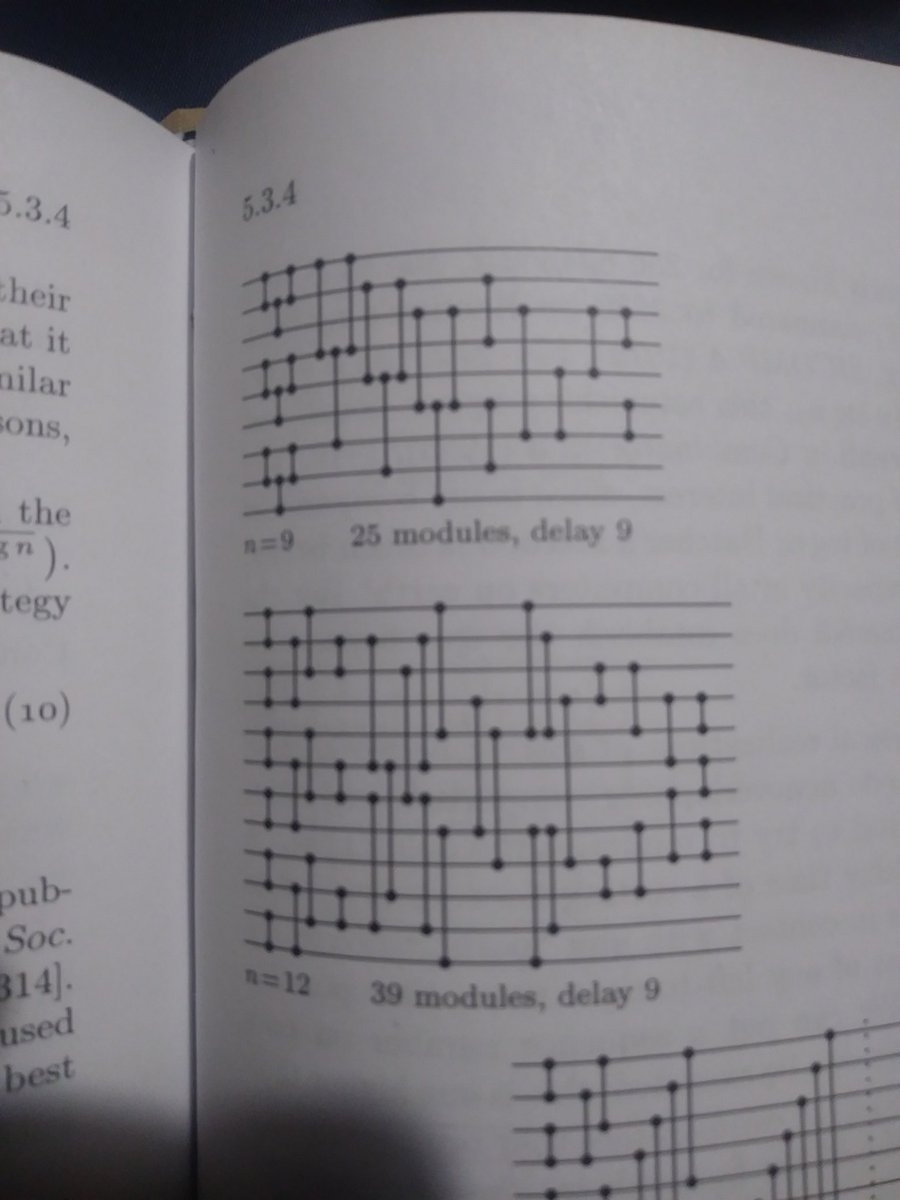So, with straight-line code of 39 CMPX instructions it is possible to sort a collection of 12 objects without any need for loops, conditional branching, or any other form of control flow. This is especially useful when programming a GPU, where control flow is toxic for performance.

I proceeded to transcribe the sorting network from the above diagram into CUDA code. As a mere mortal, I was not totally convinced that I’d copied it flawlessly, so resorted to building a test to verify the correctness of the transcribed network. Preferring to do this in a high-level language such as Python, I resorted to my usual tricks of writing a single file which is valid in two languages and incorporating it into the source code by means of one innocuous line: #include “sorting_network.py”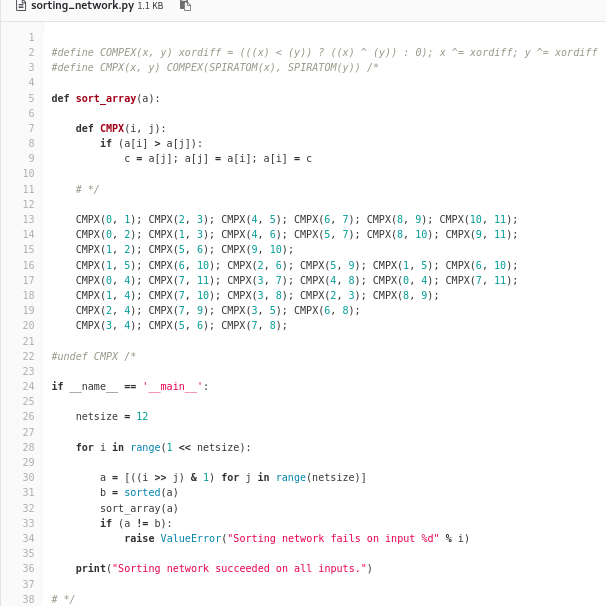(If you think this is bad, people have done much worse…)

Examining the Python component of the code, you may notice that it only tests the 2^12 different binary sequences, rather than the 12! different totally ordered sets. It is a general property of comparator networks that it suffices to only test binary sequences to prove that the network can sort arbitrary sequences; this is known as the 0-1 principle.

### Batcher’s bitonic mergesort

What is the minimum number of CMPX gates necessary to sort n objects? And what is the minimum circuit depth? The naive algorithm of bubble sort shows that a gate-count of O(n^2) and a circuit depth of O(n) are both attainable. Similarly, the gate-count must be at least the binary logarithm of n! (as with any comparison-based sorting algorithm) which gives a lower bound of Ω(n log n) for the gate-count and Ω(log n) for the depth.

Batcher found a recursive construction of sorting networks with a depth of ½k(k+1), where k is the ceiling of the binary logarithm of n, and each layer has ½n comparators. This is achieved by firstly Batcher-sorting the initial and final halves of the sequence, followed by interleaving them (diagram by User:Bitonic from Wikipedia):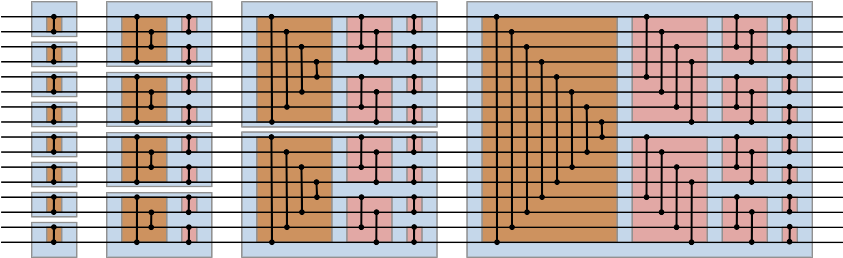The correctness of the algorithm follows from the aforementioned 0-1 principle. By the inductive hypothesis, it suffices to examine the rightmost blue box and suppose that the two halves of the input are correctly sorted, in which case the input would resemble:

[n/2 – m zeroes] [m ones] | [l zeroes] [n/2 – l ones]

The only ‘cross-lane’ operations are the comparators in the brown box. If l is no greater than m, the result of this is the following:

[n/2 – m zeroes] [m – l ones] [l zeroes] | [n/2 ones]

and otherwise we get the complementary arrangement:

[n/2 zeroes] | [m ones] [l – m zeroes] [n/2 – l ones]

Concentrating only on the non-constant half, our task is reduced to the simpler problem of sorting a binary sequence which switches at most twice between a run of zeroes and a run of ones. We can split the effect of the pink box into two modules: one which reverses one of the two halves (we get to decide which half!), followed by one which behaves identically to a brown box. Observe that, as before, one of the two halves of the pink box must therefore be constant, and the other must again be a binary sequence which switches at most twice. By induction, the result follows.

Owing to the low depth, simplicity, and efficiency, Batcher’s bitonic mergesort is often used for sorting large lists on GPUs.

### Beyond Batcher

But is the bitonic mergesort optimal? The circuit above takes 80 comparators to sort 16 inputs, whereas the best circuit in Knuth takes only 60 comparators (again with a depth of 10). It’s not even optimal for depth, as the next page of Knuth has a 61-comparator sorting network with a depth of 9.

What about asymptotics? The bitonic mergesort gives an upper bound on the depth of O((log n)^2) and basic information theory gives a lower bound of Ω(log n).

The next surprise was when Szemeredi, Komlos and Ajtai proved that the lower bound is tight: they exhibited a construction of sorting networks of optimal depth O(log n). As you can imagine from Szemeredi’s background in combinatorics and extremal graph theory, the construction relies on a family of graphs called expanders.

A simplified version of the construction (by Paterson, 1990) is described here. The original paper provides explicit constants, showing that a depth ~ 6100 log(n) is possible, compared with ~ ½ log(n)^2 for Batcher’s bitonic mergesort. In other words, the threshold for switching from bitonic mergesort to Paterson’s variant of AKS occurs when n is approximately 2^12200.

A further improvement by Chvatal reduces the asymptotic constant from 6100 to 1830, and actually provides an explicit (non-asymptotic) bound: provided n ≥ 2^78, there is a sorting network of depth 1830 log(n) − 58657. This reduces the crossover point to exactly n ≥ 2^3627. As Knuth remarked, this is still far greater than the number of atoms in the observable universe, so the practical utility of the AKS sorting algorithm is questionable.

Interestingly, this is not the first time there has been an asymptotically impressive algorithm named AKS after its authors: a set of three Indian Institute of Technology undergraduates {Agrawal, Kayal, Saxena} found the first unconditional deterministic polynomial-time algorithm for testing whether an n-digit number is prime. This O(n^(6+o(1)) algorithm tends not to be used in practice, because everyone believes the Generalised Riemann Hypothesis and its implication that the O(n^(4+o(1)) deterministic Miller-Rabin algorithm is correct.

Posted in Uncategorized | 2 Comments

## Eurozone’s Lemma

David Davis has proposed two geopolitical ideas:

• For Northern Ireland to have dual EU/UK status;
• For there to be a 10-mile ‘trade buffer zone’ between Northern Ireland and the Republic of Ireland.

The second is more interesting from a mathematical perspective: the 10-mile buffer zone means that (the closures of) Northern Ireland and the Republic of Ireland are disjoint compact subsets of a normal topological space. By Urysohn’s Lemma, this means that there exists a continuous function$f : Ireland \rightarrow [0, 1]$ such that$f$ is identically 0 on Northern Ireland and identically 1 on the Republic of Ireland.

The proof of this proceeds as follows:

• By taking closures, assume without loss of generality that NI and ROI are both closed and disjoint (the interior 10-mile buffer zone is not considered to belong to either).
• Define U(1) and V(0) to be the complements of NI and ROI, respectively. These are overlapping open sets, whose intersection is the buffer zone.
• For each$k \in \{1, 2, 3, \dots \}$:
• For each dyadic rational$r \in (0, 1)$ with denominator$2^k$ and odd numerator:
• Let$q = r - 2^{-k}$ and$s = r + 2^{-k}$, so$q,r,s$ are adjacent;
• By appealing to the normality of Ireland, let U(r) and V(r) be two disjoint open sets containing the complements of V(q) and U(s), respectively.
• Now we have disjoint open sets U(r) and V(r) for each dyadic rational r, such that the U(r) form an ascending chain of nested spaces.
• Define$f(x) := \inf \{ r : x \in U(r) \}$ (where the infimum of an empty set is taken to be 1).

With this interpolating function$f$, it is easy to take convex combinations of EU and UK standards. For example, a road sign at a point x must be stated in ‘lengths per hour’, where one length is exactly 1 + 0.609344(1 – f(x)) kilometres.

Posted in Uncategorized | Leave a comment

## Royal Wedding and Polymath16

Congratulations to Meghan Markle and Prince Harry on what is undoubtedly the most energetic Royal Wedding!

In other news, following on from Aubrey de Grey’s 5-chromatic unit-distance graph, there has been an effort to study the algebraic structure of the graphs. Specifically, viewing the vertices as points in the complex plane, one can ask what number fields contain the vertices of the unit-distance graphs.

In particular, it was noted that both Moser’s spindle and Golomb’s graph, the smallest examples of 4-chromatic unit-distance graphs, lie in the ring$\mathbb{Z}[\omega_1, \omega_3]$, where$\omega_t$ is a complex number with real part$1 - \frac{1}{2t}$ and absolute value 1. Ed Pegg Jr produced a beautiful demonstration of this: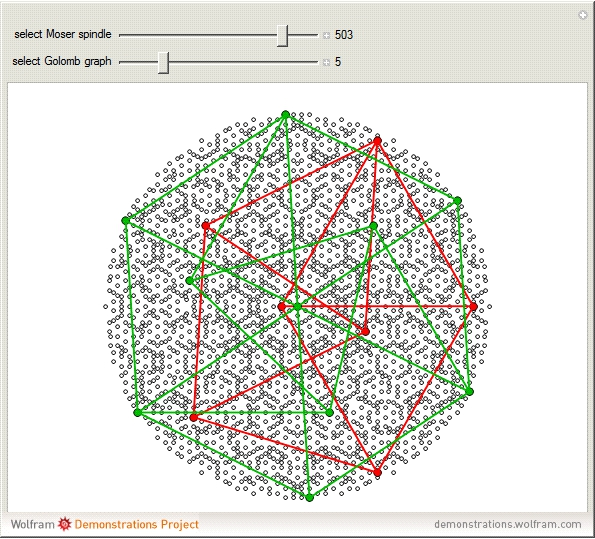Philip Gibbs showed that the entire ring, and consequently all graphs therein, can be coloured by a homomorphism to a four-element group. Consequently, Ed Pegg’s hope that the large unit-distance graph above is 5-chromatic was doomed to fail — but that is not too much of a worry now that we have de Grey’s 5-chromatic graph.

Several of Marijn Heule’s 5-chromatic graphs lie in$\mathbb{Z}[\omega_1, \omega_3, \omega_4]$. Apparently both this ring and$\mathbb{Z}[\omega_1, \omega_3, \omega_4, \omega_7]$ have homomorphic 5-colourings, so we cannot find a 6-chromatic unit-distance graph lying in either of these rings.

Incidentally, the record is a 610-vertex example, again due to Heule: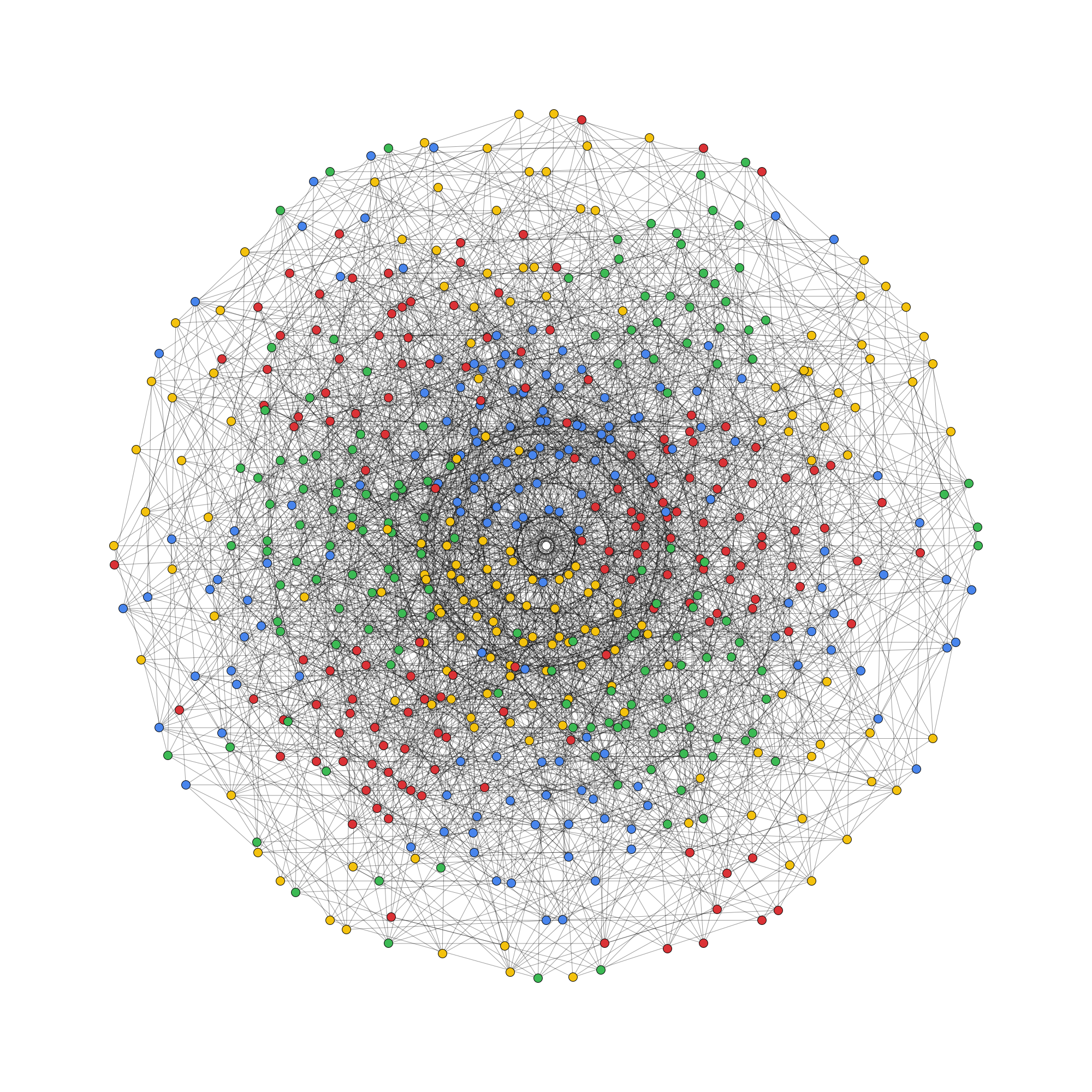Posted in Uncategorized | 1 Comment

## Assorted news

This last week has been very exciting. On Thursday, I gave a talk in absentia at the 13th Gathering for Gardner on the topic of artificial life (thanks go to Dave Greene and Tom Rokicki for playing the slides and audio recording). Meanwhile, this happened:

### Chromatic number of the plane is at least 5

Aubrey de Grey (!!!) has found a unit-distance graph with 1567 vertices and a chromatic number of 5. This implies that the chromatic number of the plane is between 5 and 7, the latter bound obtainable from 7-colouring the cells of an appropriately-sized hexagonal lattice. Before, the best lower bound was 4 (from the Moser spindle).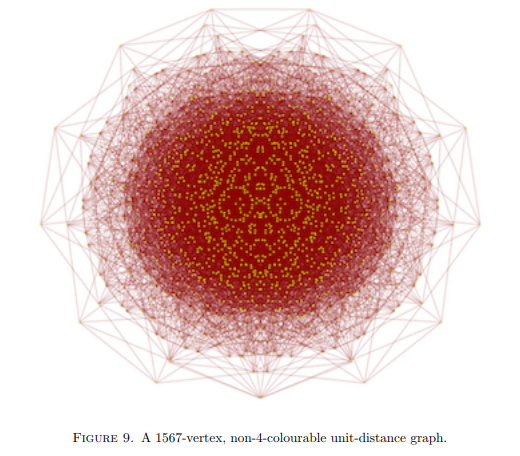There is now a polymath project to reduce the number of vertices from 1567. Marijn Heule, whom I mentioned last time for providing me with the incremental SAT solver used to find Sir Robin, has already reduced it down to 874 vertices and 4461 edges.

### EGMO results

The results of the European Girls’ Mathematical Olympiad have been published. The UK came third worldwide, just behind Russia and the USA. Moreover, Emily Beatty was one of five contestants to gain all of the available marks, and apparently the first UK contestant to do so in any international mathematical competition since 1994.

It appears that EGMO has been following the example of the Eurovision Song Contest in determining which countries are European, rather than actually verifying this by looking at a map. Interesting additions include the USA, Canada, Saudi Arabia, Israel, Australia, Mongolia, Mexico and Brazil. The list of participating countries states that there are 52 teams, of which 36 are officially European (and a smaller number still are in the EU).

Restricting to the set of countries in the European Union, the UK won outright (three points ahead of Poland), which was the last opportunity to do so before the end of the Article 50 negotiations. Hungary and Romania put in a very strong performance, as expected.

Posted in Uncategorized | 2 Comments

## Cubical Type Theory

Previously, we discussed Homotopy Type Theory, which is an alternative foundation of mathematics with several advantages over ZFC, mainly for computer-assisted proofs. It is based on Martin-Löf’s intuitionistic type theory, but with the idea that types are spaces, terms are points within that space, and that A = B is the space of paths between A and B (viewed as points in a universe U). To accomplish this, recall that there are two extra modifications beyond intuitionistic type theory:

• Voevodsky’s univalence axiom states that equivalent types are equal, and more specifically that the space of equivalences between two types is equivalent to the space of paths between two types;
• The introduction of higher inductive types which define not only their elements (vertices) but also paths, 2-cells (paths between paths), and so forth.

An advantage of intuitionistic type theory is that the lack of axioms mean that all proofs of constructive. Including the univalence axiom breaks this somewhat, which is why people have been searching for a way to adapt type theory to admit univalence not as an axiom, but as a theorem.

Cubical type theory was introduced as a constructive formulation which includes univalence and has limited support for certain higher inductive types. A recent paper by Thierry Coquand (after whom the automated theorem-prover Coq is named), Simon Huber, and Anders Mörtberg goes further towards constructing higher inductive types in cubical type theory.

Posted in Uncategorized | Leave a comment

## Diamonds

Tim Hutton recently presented me with a marvellous 3D-printed-graphite set of triakis truncated tetrahedra. These have a natural interpretation as the Voronoi cells of a diamond: the shapes that would form if you gradually enlarged the atoms until they tessellate the ambient space.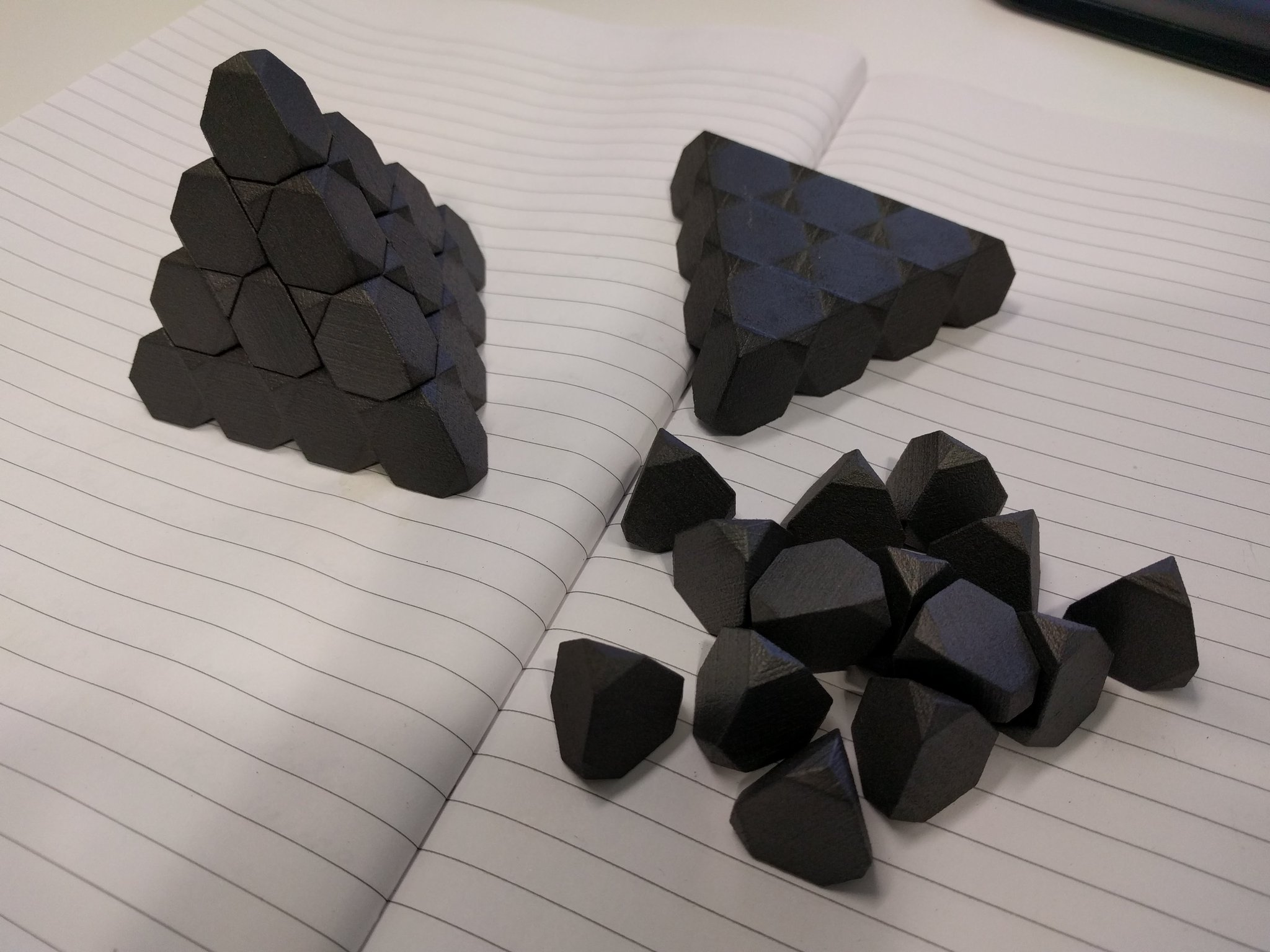He also produced a video describing the relationship between the Voronoi cells of the diamond and related lattices.

### Hyperdiamonds

Mathematically, the set of centres of atoms in a diamond form a structure called$D_3^+$. It has a natural n-dimensional generalisation, which can be viewed as follows:

• Take the integer lattice$\mathbb{Z}^n$;
• Partition it into two subsets, called E and O, consisting of the points whose coordinate-sums are even and odd, respectively;
• Translate the set O such that it contains the point (½, ½, ½, …, ½), and leave E where it is.

This construction makes it adamantly* clear that the ‘hyperdiamond’ has the same density as the integer lattice, and therefore the Voronoi cells have unit volume. A lattice with this property (such as the hyperdiamonds of even dimension) is described as unimodular.

*bizarrely, this word has the same etymology as ‘diamond’, namely the Greek word adamas meaning ‘indestructible’. I often enjoy claiming that my own given name is also derived from this same etymology.

In the special case where n = 8, this is the remarkable E8 lattice, which Maryna Viazovska proved is the densest way to pack spheres in eight-dimensional space. One could imagine that if beings existed in eight-dimensional space, they would have very heavy diamond engagement rings! It is an even unimodular lattice, meaning that the inner product of any two vectors is an even integer, and is the unique such lattice in eight dimensions. They can only exist in dimensions divisible by 8, and are enumerated in A054909.

If n = 16, the hyperdiamond gives one of two even unimodular 16-dimensional lattices, the other being the Cartesian product of E8 with itself. These two lattices have the same theta series (sequence giving the number of points at each distance from the origin), but are nonetheless distinct. If you take the quotients of 16-dimensional space by each of these two lattices, you get two very similar (but non-isometric) tori which Milnor gave as a solution to the question of whether two differently-shaped drums could produce the same sound when reverberated.

John Baez elaborates on the sequence of ‘hyperdiamonds’, together with mentioning an alternative three-dimensional structure called the triamond, here.

### Niemeier lattices

There are 24 even unimodular lattices in 24 dimensions, the so-called Niemeier lattices, of which the most famous and stunning is the Leech lattice. This was also proved by Viazovska and collaborators to be the densest sphere packing in its dimension. Unlike the hyperdiamonds, however, the Leech lattice is remarkably complicated to construct.

Whereas the shortest nonzero vectors in the Leech lattice have squared norm of 4, the other 23 Niemeier lattices have roots (elements of squared norm 2). The Dynkin diagrams of these root systems identify the lattices uniquely, and conversely all Dynkin diagrams with certain properties give rise to Niemeier lattices.

The 23 other Niemeier lattices correspond exactly to the equivalence classes of deep holes in the Leech lattice, points at a maximum distance from any of the lattice points.

Posted in Uncategorized | 5 Comments

## Happy Noethday

I’ve just realised, with only two minutes to go, that it’s the 136th birthday of the great mathematician Emmy Noether.

Naturally, you are probably already aware of many of her contributions to mathematics and theoretical physics, including Noetherian rings and Noether’s theorem. I was pleasantly surprised to hear that she also laid the foundations of homology theory, which is especially pertinent as my research in topological data analysis is built upon these ideas.For more information, see the wonderful Wikipedia article entitled List of things named after Emmy Noether. It appears that it is incomplete, omitting the Emmy Noether Society of Cambridge.

Finally, even at the age of five her surname anticipated the result of the Michelson-Morley experiment which proved that there was, in fact, no ether…

Posted in Uncategorized | Leave a comment

## Serge Vlăduţ : Lattices with exponentially large kissing numbers

Conway and Sloane’s excellent tome, Sphere Packings, Lattices and Groups, is now due a fourth edition. Maryna Viakovska’s proofs of optimality of the E8 and Leech lattices should go in the fourth edition as well…

Posted in Uncategorized | Leave a comment